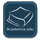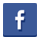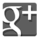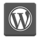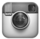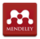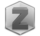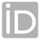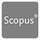You are in:Home/Publications

### Dr. Abdelhameed Mohamed Abdelhameed Nagy :: Publications:

Research Fields
 Mathematics
 Number of publications is 28
 Title 1-Uniform asymptotic stability of a Logistic model with feedback control of fractional order and nonstandard finite difference schemesAbstract 2-Finite-Time Stability for Caputo–Katugampola Fractional-Order Time-Delayed Neural NetworksAbstract 3-Finite‐Time Stability of Linear Caputo‐Katugampola Fractional‐Order Time Delay Systems 4-Nonstandard Finite Difference Scheme For The Fractional Order Salmonella Transmission ModelAbstract 5-An accurate numerical technique for solving two-dimensional time fractional order diffusion equationAbstract 6-New Operational Matrix for Solving Multiterm Variable Order Fractional Differential EquationsAbstract 7-Numerical simulations for a variable order fractional cable equationAbstract 8-On the Numerical Treatment of a Coupled Nonlinear System of Fractional Differential EquationsAbstract 9-Numerical solution of time fractional nonlinear Klein–Gordon equation using Sinc–Chebyshev collocation methodAbstract 10-New Spectral Second Kind Chebyshev Wavelets Scheme for Solving Systems of Integro-Differential Equations 11-New Operational Matrix for Solving Multi-Term Variable Order Fractional Differential EquationsAbstract 12-Comparative Studies for Different Image Restoration MethodsAbstract 13-Non-Standard Finite Difference Schemes for Solving Variable-Order Fractional Differential EquationsAbstract 14-On the numerical solution of space fractional order diffusion equation via shifted Chebyshev polynomials of the third kind 15-Computational methods for the fractional optimal control HIV infection 16-Solving Time-Fractional Order Telegraph Equation Via Sinc–Legendre Collocation MethodAbstract 17-Numerical approach for solving space fractional order diffusion equations using shifted Chebyshev polynomials of the fourth kind 18-Second kind shifted Chebyshev polynomials for solving space fractional order diffusion equationAbstract 19-Solving Volterra integro-differential equations by variable stepsize block BS methods: Properties and implementation techniquesAbstract 20-A new mesh selection strategy with stiffness detection for explicit Runge–Kutta methodsAbstract 21-An Efficient Method for Solving Fractional Hodgkin-Huxley ModelAbstract 22-The MATLAB code bvptwp.m for the numerical solution of two point boundary value problemsAbstract 23-Numerical solution of two-sided space fractional wave equation using finite difference methodAbstract 24-Numerical solution of fractional wave equation using Crank-Nicholson method 25-Stiffness detection strategy for explicit Runge Kutta methodsAbstract 26-Efficient dynamical systems methods for solving singularly perturbed integral equations with noiseAbstract 27-Numerical studies on dynamical systems method for solving ill-posed problems 28-Two Dimensions Gravity Inverse Problem Using Adaptive Pruning L-Curve Technique